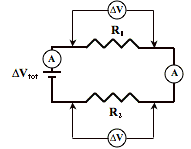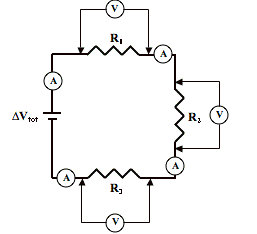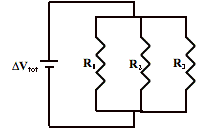# Electricity: Electric Circuits

## Electric Circuits: Problem Set

#### Problem 1:

Over the course of an 8 hour day, 3.8x104 C of charge pass through a typical computer (presuming it is in use the entire time). Determine the current for such a computer.

#### Problem 2:

The large window air conditioner in Anita Breeze's room draws 11 amps of current. The unit runs for 8.0 hours during the course of a day. Determine the quantity of charge that passes through Anita's window AC during these 8.0 hours.

#### Problem 3:

Determine the amount of time that the following devices would have to be used before 1.0x106 C (1 million Coulombs) of charge passes through them.
a. LED night light (I=0.0042 A)
b. Incandescent night light (I=0.068 A)
c. 60-Watt incandescent light bulb (I=0.50 A)
d. Large bathroom light fixture (I=2.0 A)

#### Problem 4:

The heating element of an electric toaster is typically made of nichrome wire (an alloy of nickel and chromium). As current passes through the wires, the wires heat up, thus toasting the toast. Estimate the overall resistance of a heating element which is 220 cm long and consists of nichrome wire with a diameter of 0.56 mm. The resistivity of nichrome is 110x10-8 Ω•m.

#### Problem 5:

Determine the overall resistance of a 100-meter length of 14 AWA (0.163 cm diameter) wire made of the following materials.
a. copper (resistivity = 1.67x10-8 Ω•m)
b. silver (resistivity = 1.59x10-8 Ω•m)
c. aluminum (resistivity = 2.65x10-8 Ω•m)
d. iron (resistivity = 9.71x10-8 Ω•m)

#### Problem 6:

A power saw at the local hardware store boasts of having a 15-Amp motor. Determine its resistance when plugged into a 110-Volt outlet.

#### Problem 7:

A coffee cup immersion heater utilizes a heating coil with a resistance of 8.5 Ω. Determine the current through the coil when operated at 110 V.

#### Problem 8:

Defibrillator machines are used to deliver an electric shock to the human heart in order to resuscitate an otherwise non-beating heart. It is estimated that a current as low as 17 mA through the heart is required to resuscitate. Using 100,000 Ω as the overall resistance, determine the output voltage required of a defibrillating device.

#### Problem 9:

A stun gun or TASER is designed to put out a few seconds worth of electric pulses that impress a voltage of about 1200 V across the human body. This results in an average current of approximately 3 mA into a human body. Using these figures, estimate the resistance of the human body.

#### Problem 10:

Determine the amount of electrical energy (in J) used by the following devices when operated for the indicated times.
a. Hair dryer (1500 W) - operated for 5 minutes
b. Electric space heater (950 W) - operated for 4 hours
c. X-Box video game player (180 W) - operated for 2 hours
d. 42-inch LCD television (210 W) - operated for 3 hours

#### Problem 11:

Alfredo deDarke sleeps with a 7.5-Watt night light bulb on. He turns it on before getting in bed and turns it off 8 hours later.
a. Determine the amount of energy used during one evening in units of kiloWatt•hours.
b. Electrical energy costs 13 cents/kW•hr where Alfredo lives. Determine the annual (365 days) cost of this practice of using a 7.5-Watt night light.
c. Determine the annual savings if Alfredo replaced his 7.5-Watt incandescent night light by a 0.5-Watt LED night light.

#### Problem 12:

Having recently lost her job, Penny Penching is looking for every possible means of cutting costs. She decides that her 4.0-Watt clock radio alarm does not need to be on for 24 hours every day since she only needs it for waking up after her average 8-hour sleep. So she decides to plug it in before going to sleep and to unplug it when waking. Penny pays 12 cents per kiloWatt•hour for her electricity. How much money is Penny able to save over the course of a month (31 days) with her new alarm clock usage pattern?

#### Problem 13:

The power of a 1.5-volt alkaline cell varies with the number of hours of operation. A brand new D-cell can deliver as much as 13 A through a copper wire connected between terminals. Determine the power of a brand new D-cell.

#### Problem 14:

A central air conditioner in a typical American home operates on a 220-V circuit and draws about 15 A of current.
a. Determine the power rating of such an air conditioner.
b. Determine the energy consumed (in kW•hr) if operated for 8 hours per day.
c. Determine the monthly cost (31 days) if the utility company charges 13 cents per kW•hr.

#### Problem 15:

During the Christmas season, Sel Erbate uses the equivalent of 45 strings of 100 mini-bulbs to light the inside and outside of his home. Each 100-bulb string of lights is rated at 40 Watts. The average daily usage of the strings is 7 hours. The lights are used for approximately 40 days during the holiday season.
a. Determine the resistance of each string of lights. Each is powered by 110-volt outlet.
b. Determine the energy consumed (in kW•hr) by the lights over the course of 40 days.
c. If Sel pays 12 cents/kW•hr for electrical energy, then what is the total cost of Christmas lighting for a single season?

#### Problem 16:

A 3-way light bulb for a 110-V lamp has two different filaments and three different power ratings. Turning the switch of the lamp toggles the light from OFF to low (50 W) to medium (100 W) to high (150 W) brightness. These three brightness settings are achieved by channeling current through the high resistance filament (50 W), the low resistance filament (100 W) or through both filaments. Determine the resistance of the 50 W and the 100 W filaments.

#### Problem 17:

Compare the resistance of a 1.5-Amp interior light bulb of a car (operating off a 12-V battery) to the resistance of a 100-Watt bulb operating on a 110-volt household circuitry.

#### Problem 18:

An overhead high voltage (4.0x105 V) power transmission line delivers electrical energy from a generating station to a substation at a rate of 1500 MW (1.5x109 W). Determine the resistance of and the current in the cables.

#### Problem 19:

The UL panel on the bottom of an electric toaster oven indicates that it operates at 1500 W on a 110 V circuit. Determine the electrical resistance of the toaster oven.

#### Problem 20:

Determine the equivalent resistance of a 6.0 Ω and a 8.0 Ω resistor if …
a. … connected in series.
b. … connected in parallel.

#### Problem 21:

Two resistors with resistance values of 6.0 Ω and 8.0 Ω are connected to a 12.0-volt source. Determine the overall current in the circuit if the resistors are …
a. … connected in series.
b. … connected in parallel.

#### Problem 22:The series circuit at the right depicts two resistors connected to a voltage source. The voltage source (ΔVtot) is a 48-V source and the resistor values are 6.4 Ω (R1) and 3.9 Ω (R2).
a. Determine the equivalent resistance of the circuit.
b. Determine the current in the circuit.
c. Determine the voltage drop across each individual resistor.

#### Problem 23:Voltmeters can be used to determine the voltage difference between two points on a circuit. An ammeter can be used to determine the current at any given location on a circuit. The circuit at the right is powered by a 12.0-volt battery and utilizes two voltmeters and two ammeters to measure voltage drops and currents. The resistor values are 1.28 Ω (R1) and 3.85 Ω (R2). Determine the ammeter readings and voltmeter readings.

#### Problem 24:The series circuit at the right depicts three resistors connected to a voltage source. The voltage source (ΔVtot) is a 110-V source and the resistor values are 7.2 Ω (R1), 6.2 Ω (R2) and 8.6 Ω (R3).
a. Determine the equivalent resistance of the circuit.
b. Determine the current in the circuit.
c. Determine the voltage drops across each individual resistor.

#### Problem 25:Voltmeters can be used to determine the voltage across two points on a circuit. An ammeter can be used to determine the current at any given location on a circuit. The circuit at the right is powered by a 60.0-volt power source and utilizes three voltmeters and three ammeters to measure voltage drops and currents. The resistor values are 10.3 Ω (R1), 15.2 Ω (R2) and 12.8 Ω (R3). Determine the ammeter readings and voltmeter readings.

#### Problem 26:

A circuit powered by a 12.0-volt battery is comprised of three identical resistors in series. An ammeter reading reveals a current of 0.360 A. Determine the resistance values of the resistors and the voltage drops across the resistors.

#### Problem 27:

A 4.5-volt series circuit consists of two resistors. Resistor A has three times the resistance as resistor B. An ammeter records a current of 160 mA of current. Determine the resistance values of resistors A and B.

#### Problem 28:

A 9.00-volt battery is used to power a series circuit with a 2.50 Ω and a 3.50 Ω resistor. Determine the power rating of each resistor and the total power of the circuit.

#### Problem 29:

Determine the equivalent resistance of a parallel arrangement of two resistors with resistance values of …
a. … 8.0 Ω and 8.0 Ω
b. … 5.0 Ω and 5.0 Ω
c. … 5.0 Ω and 8.0 Ω
d. … 5.0 Ω and 9.2 Ω
e. … 5.0 Ω and 27.1 Ω
f. … 5.0 Ω and 450 Ω

#### Problem 30:The parallel circuit at the right depicts two resistors connected to a voltage source. The voltage source (ΔVtot) is a 12-V source and the resistor values are 6.4 Ω (R1) and 3.9 Ω (R2).
a. Determine the equivalent resistance of the circuit.
b. Determine the current in each branch resistor.
c. Determine the total current in the circuit.

#### Problem 31:The parallel circuit at the right depicts three resistors connected to a voltage source. The voltage source (ΔVtot) is a 110.-V source and the resistor values are 15.4 Ω (R1), 21.9 Ω (R2) and 11.7 Ω (R3).
a. Determine the equivalent resistance of the circuit.
b. Determine the current in each branch resistor.
c. Determine the total current in the circuit.

#### Problem 32:

Voltmeters can be used to determine the voltage across two points on a circuit. An ammeter can be used to determine the current at any given location on a circuit. The circuit below is powered by a 24.0-volt power source and utilizes four voltmeters and three ammeters to measure voltage drops and currents.The resistor values are 54.5 Ω (R1), 31.7 Ω (R2) and 48.2 Ω (R3). Determine the ammeter readings and voltmeter readings.

#### Problem 33:

A 9.00-volt battery is used to power a parallel circuit with a 2.50 Ω and a 3.50 Ω resistor. Determine the power rating of each resistor and the total power of the circuit.

#### Problem 34:

Cullen Ary's family loves to cook. According to Cullen's friends, they have every imaginable kitchen gadget that exists. One Sunday afternoon, they have a cooking party in which every member of the family participates. They get out the following small appliances, plug them in and turn them on.

Mixer (81 Ω)
Crockpot (62 Ω)
Juicer (43 Ω)
Blender (21 Ω)
Electric Fondue (16 Ω)
Wok (12 Ω)
Rotisserie (7.5 Ω)
Deep-fat fryer (7.0 Ω)

The resistance values for each appliance is listed in parenthesis. Each appliance is plugged in to 110-volt receptacles which are wired in parallel on the same circuit. The circuit is protected by a 20-amp circuit breaker.

a. Determine the overall current on the circuit with the mixer and crockpot operating.
b. Determine the overall current on the circuit with the mixer, crockpot and juicer operating.
c. Determine the overall current on the circuit with the mixer, crockpot, juicer and blender operating.
d. Determine the overall current on the circuit with the mixer, crockpot, juicer, blender and electric fondue operating.
e. Determine the overall current on the circuit with the mixer, crockpot, juicer, blender, electric fondue, and wok operating.
f. Determine the overall current on the circuit with the mixer, crockpot, juicer, blender, electric fondue, wok, and rotisserie operating.
g. Determine the overall current on the circuit with the mixer, crockpot, juicer, blender, electric fondue, wok, rotisserie, and the deep-fat fryer operating.
h. At what point in the progression of turning on appliances will the circuit become overloaded and the circuit breaker interrupt the circuit.